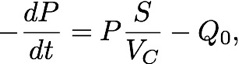The term ultra-high vacuum vacuum stands for vacuum with a rest gas pressure of around 10-9 mbar and below. There are couple of essential physical ideas you need to know when you are working with such low pressures. A very important aspect is that a low-pressure rest gas in the vacuum recipient does not flow and behave in the way we are used to from gasses at ambient pressure.

It is very instructive to calculate two quantities for typical UHV pressures: (1) the mean distance the molecules in the system travel before they bump into each other (the so-called mean free path) and (2) the rate at which molecules impinge on a surface in the vacuum system. Both can be calculated by using kinetic gas theory.

The mean free path λ of the molecules is

where ξ is the molecular diameter, P is the pressure of the gas and T is the temperature. The usual units for the pressure in vacuum technology are torr or mbar (1 torr = 1.3332 mbar = 133.32 Pascal). By the way the pressure enters in this equation, we can directly see that the mean free path is going to be very long in UHV. You find that  λ for a gas (nitrogen) at ambient conditions is in the order of nm. But since the pressure under UHV conditions is some 12 orders of magnitude lower, the mean free path has to be in the order of km. This means that it is much more likely for the rest gas molecules to hit the walls of the the UHV vessel than to hit each other! It has also consequences for pumping: at ambient pressure we are used to the fact that pressure differences propagate quickly in a gas (like in sound waves or when using a straw for drinking). For UHV this is simply not true anymore. It is the individual molecules we have to think about and, in fact, pumps based on the usual gas flow idea fail to produces UHV. The gas flow is usually divided in two regimes. The flow under ambient conditions is called viscous and the flow under UHV conditions is called molecular.

(2) The rate of impinging molecules R on a surface is

where P is the pressure of the rest gas and M the molecular mass in units of the atomic mass constant. For a pressure of 10-6 mbar (i.e. high vacuum but not yet UHV) and a temperature of 300K we findAs an order of magnitude value, a surface has 1015 atoms per square centimetre. This means that if every rest-gas molecule at the above conditions sticks to a surface the latter will only

stay clean for a second or so. If we are not willing to tolerate more  than, say, a percent of contaminating rest-gas molecules on the surface then the pressure has to be in the UHV region.

Finally, it is useful to describe the pumping of a UHV system a bit more formally. The objective of vacuum technology is to remove gas from the recipient. A flow of gas can be defined in terms of the volumetric flow dV / dt or, more usefully, the flow rate Q defined as

basic physics behind UHVFlow rates are measured in Pa m3s-1 or similar units (one often finds torr for pressure and litres for volume).

Using the ideal gas equation, we obtainso this does indeed corresponds to a flow of particles. The flow rate can be used to describe different situations, such as the flow through a tube connecting vacuum system and pump, the removal of particles from vacuum system or an incoming flow of particles due to a leak in the sealing of the system.

The process of evacuating the system can be described aswhere VC is the volume of the vacuum chamber, Q0 some incoming flow of particles discussed below and S the pumping speed. S is measured in m3s-1 but for commercially available pumps it is most frequently quoted in litres per second (ls-1). To see how the pump-down of the system proceeds, we first consider the  limit of this equation when Q0 is so small that we can neglect it. In this case, the pumping equation is solved bywhere P0 is the starting pressure. Such exponential behaviour is indeed found in the beginning of the pumping process but unfortunately it does not continue, even in the case of Q0 = 0. The reason is that the pumping speed of a vacuum pump depends on the pressure. In fact, apart from the pumping speed, vacuum pumps are also characterised by the lowest achievable pressure (the pressure where S approaches zero) and in order to achieve UHV, it is usually necessary to use a combination of pumps with different characteristics, frequently operated in series.